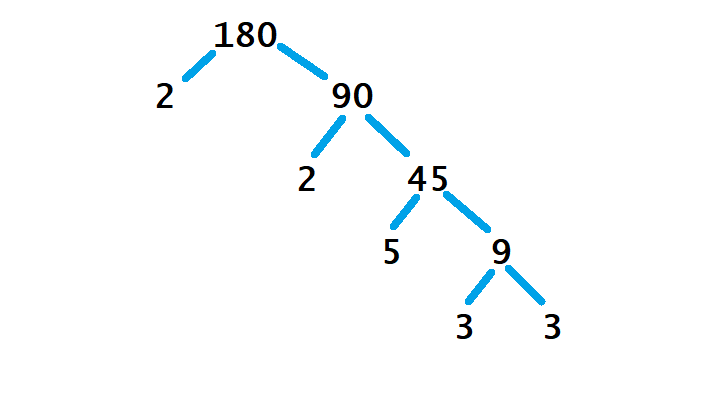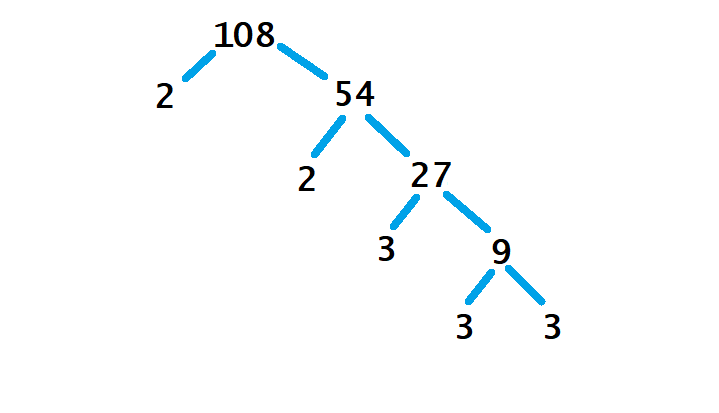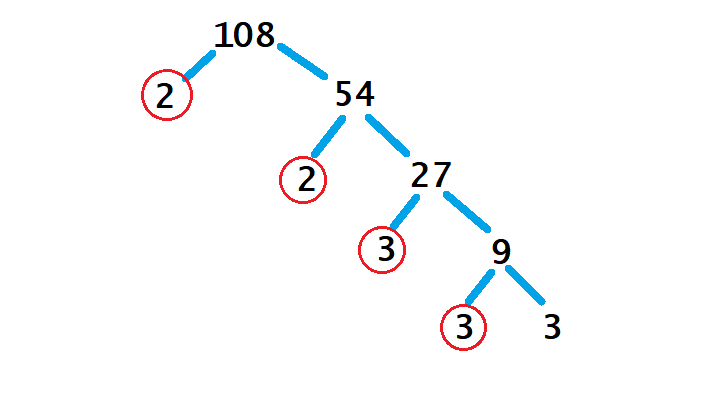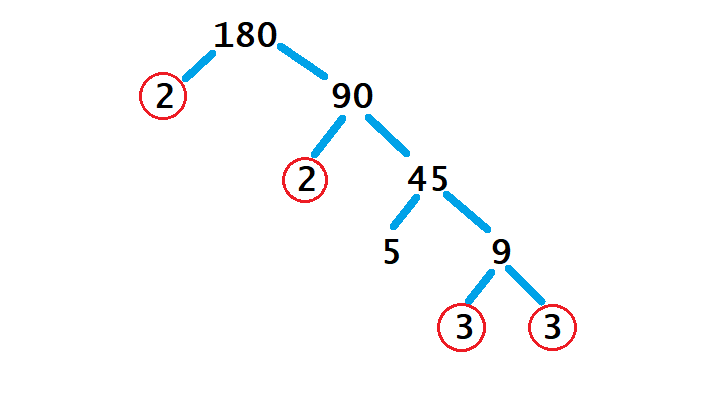# EdPlace's Year 7 home learning maths lesson: HCF and LCM

Looking for short lessons to keep your child engaged and learning? Our experienced team of teachers have created English, maths and science lessons for the home, so your child can learn no matter where they are.  And, as all activities are self-marked, you really can encourage your child to be an independent learner.

Get them started on the lesson below and then jump into our teacher-created activities to practice what they've learnt. We've recommended five to ensure they feel secure in their knowledge - 5-a-day helps keeps the learning loss at bay (or so we think!).

Are they keen to start practising straight away? Head to the bottom of the page to find the activities.

Now...onto the lesson!

Key Stage 3 Statutory Requirements for Maths
Year 7  students should be able to use the concepts and vocabulary of prime numbers, factors, multiples, common factors, common multiples, highest common factor, lowest common multiple, [and] prime factorisation.

This guide will explain how to cover highest common factor (HCF) and lowest common multiple (LCM). There are many definitions that need remembering, so this will include ways of understanding the key vocabulary, as well as the methods required to find LCM and HCF.   We're confident that if you follow this step-by-step approach together, your child will be able to:

1) Write a number as a product of prime factors.

2) Find the highest common factor of two numbers.

3) Work-out the lowest common multiple of a pair of numbers.

## Factors and Multiples

The best way to introduce this topic is to define “factor” and “multiple”, as the two are often confused.

Factors are numbers that divide into a given number. For example, factors of 20 are 1, 2, 4, 5, 10, and 20. These numbers divide into 20 with no remainder.

Multiples of a number are answers we get when we multiply that number. (Think “multiple” and “multiply”). Another way to think of it would be: “the answers to its times table”. For example, the multiples of 20 are 20, 40, 60, 80, 100, and so on.

It is key that your child can tell the difference between factors and multiples.

## Now let us move on and discuss “prime” numbers. The definition of a prime number is a number that can only divide by itself and 1. (In other words, it only has two factors; it is not a solution in any times tables). For instance, 7 is a prime number because its only factors are 1 and 7. Meanwhile, 20 is not prime, because as we saw in Step 1, it has more than two factors.

An important point here is 1 is not a prime number. This is because it only has one factor (which is itself!), not two.

## Step 1- Factor Tree's

Once you understand prime numbers, you should be able to write a number as the product of its prime factors. This is quite a common question that you can be tested on, and sounds complicated, but all you need to do is keep dividing the number up until you reach prime numbers.

For example, if we want to write 180 as the product of prime factors, we draw a “factor tree” and split the number up by dividing it as far as we can. Every pair of “branches” represents a pair of numbers that multiply together to make the number above it.We have divided 180 as far as we can take it. The 2s, 3s, and 5 cannot have any branches added because they are prime.

So, as the product of its prime factors, 180 = 2 x 2 x 3 x 3 x 5.

## Step 2 - Highest Common Factor

This leads us to finding the highest common factor (HCF) of two numbers.

All we need to do is make two “factor trees” as we saw in Step 1, then see what numbers occur at the end of both trees.

For example, let us find the HCF of 180 and 108 by drawing both trees:All we need to do now is circle all the numbers that appear in both lists, and then multiply them.As we can see, these trees share a pair of 2s and a pair of 3s, so the HCF is 2 x 2 x 3 x 3 = 36.

## Step 3 - Lowest Common Multiple

Finally, we will look at how to find the lowest common multiple (LCM) of two numbers.

As we considered in the Factors and Multiples segment, multiples are the solutions to times tables, so the easiest way to find the LCM is to list both times tables until you reach the same number in both lists.

For example, if we want the LCM of 9 and 12:

Multiples of 9 = 9, 18, 27, 36, 45, …

Multiples of 12 = 12, 24, 36, 48, …

36 is the first number to appear in both lists, so that is the LCM.

## Step 4 - Putting it into Practice

1) Write down all the prime numbers between 1 and 10.

2) Write 84 as a product of its prime factors

3) Work out the LCM of 12 and 20

4) Work out the HCF of 24 and 36

5) Cans of lemonade are sold in packs of 6. Cans of cola are sold in packs of 4. You want the same amount of each drink. What is the minimum number of drinks you need to buy?

## Step 5 - Give it a go...

Why not test your child's understanding and see if they can tackle these activities?

All activities are created by teachers and automatically marked. Plus, with an EdPlace subscription, we can automatically progress your child at a level that's right for them. Sending you progress reports along the way so you can track and measure progress, together - brilliant!

1) The prime numbers are 2, 3, 5, 7.

2) 84 = 7 x 3 x 2 x 2

3) By listing both times tables:
12, 24, 36, 48, 60
20, 40, 60

LCM = 60

4) If we draw both factor trees we would find:
24 = 2 x 2 x 2 x 3
36 = 2 x 2 x 3 x 3
These share a pair of 2s and one 3, so if we multiply, the HCF is 2 x 2 x 3 = 12.

5) Questions like this are LCM. The lowest common multiple of 4 and 6 is 12. So you would buy 12 cans of each drink.

Keep going! Looking for more activities, different subjects or year groups?

Click the button below to view the EdPlace English, maths, science and 11+ activity library